Function Repository Resource:

# InverseSigmaConfidenceLevel

Calculate the number of standard deviations of a normal distribution that correspond to a confidence level

Contributed by: Julien Kluge
 ResourceFunction["InverseSigmaConfidenceLevel"][cl] calculates the number of standard deviations around the mean containing the given fraction of a normal distribution.

## Details and Options

ResourceFunction["InverseSigmaConfidenceLevel"] evaluates to exact outputs for exact inputs and certain values.
The associated standard deviation for a one-sided p-value can be calculated by using σ=ResourceFunction["InverseSigmaConfidenceLevel"][1-2p].

## Examples

### Basic Examples (2)

A confidence level of 0.95 spans approximately two standard deviations:

 In:=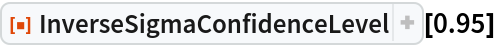Out=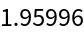A one-sided p-value of 0.05 is equivalent to around 1.64 standard deviations:

 In:=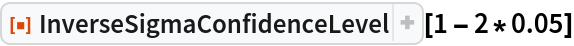Out=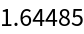### Scope (4)

Exact inputs yield exact results:

 In:=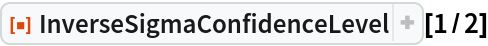Out=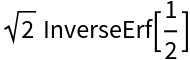In:=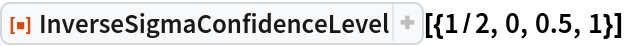Out=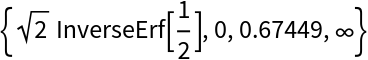InverseSigmaConfidenceLevel can be defined as the inverse CDF of the NormalDistribution with mean 0 and standard deviation 1:

 In:=Out=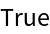InverseSigmaConfidenceLevel is the inverse to the resource function SigmaConfidenceLevel:

 In:=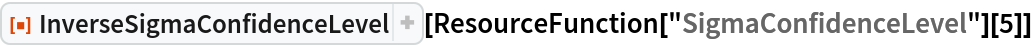Out=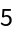In:=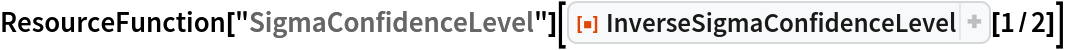Out=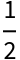### Properties and Relations (2)

Values of unity get replaced with Infinity:

 In:=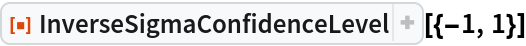Out=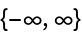Arguments containing a top-level Erf get replaced with an expression containing no Erf or InverseErf heads:

 In:=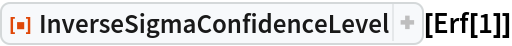Out=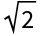### Possible Issues (1)

Values with a magnitude above one are left unevaluated:

 In:=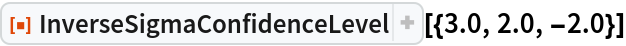Out=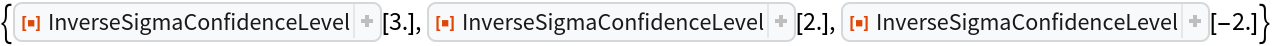### Neat Examples (1)

The Mensa organization only accepts members with an IQ test coming out in the top 2%. Calculate the lower IQ limit to pass this bar:

 In:=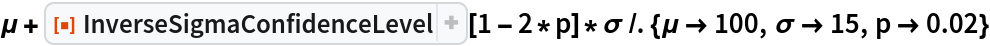Out=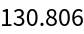Julien Kluge

## Version History

• 1.0.0 – 12 May 2020

## Author Notes

Julien Kluge

Quantum Optical Metrology; Joint Lab Integrated Quantum Sensors

Department of Physics

Humboldt-Universität zu Berlin

julien@physik.hu-berlin.de